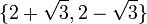# Difference between revisions of "Element structure of special linear group:SL(2,5)"

View element structure of particular groups | View other specific information about special linear group:SL(2,5)

This article gives detailed information about the element structure of special linear group:SL(2,5), which is a group of order 120.

## Conjugacy class structure

### Interpretation as special linear group of degree two

Further information: element structure of special linear group of degree two over a finite field

Nature of conjugacy class Eigenvalue pairs of all conjugacy classes Characteristic polynomials of all conjugacy classes Minimal polynomials of all conjugacy classes Size of conjugacy class (generic odd$q$) Size of conjugacy class ($q = 5$) Number of such conjugacy classes (generic odd$q$) Number of such conjugacy classes ($q = 5$) Total number of elements (generic odd$q$) Total number of elements ($q = 5$) Representative matrices (one per conjugacy class)
Scalar$\{ 1, 1 \}$ or$\{ -1,-1\}$$x^2 - 2x + 1$ or$x^2 + 2x + 1$$x - 1$ or$x + 1$ 1 1 2 2 2 2$\begin{pmatrix} 1 & 0 \\ 0 & 1 \\\end{pmatrix}$ and$\begin{pmatrix} -1 & 0 \\ 0 & -1\\\end{pmatrix}$
Not diagonal, Jordan block of size two$\{ 1, 1 \}$ or$\{ -1,-1\}$$x^2 - 2x + 1$ or$x^2 + 2x + 1$$x^2 - 2x + 1$ or$x^2 + 2x + 1$$(q^2 - 1)/2$ 12 4 4$2(q^2 - 1)$ 48 [SHOW MORE]
Diagonalizable over field:F25, not over field:F5. Must necessarily have no repeated eigenvalues.$\{ 2 + \sqrt{3}, 2 - \sqrt{3} \}$ and$\{ -2 + \sqrt{3}, -2 - \sqrt{3} \}$, where$\sqrt{3}$ is interpreted a an element of field:F25 that squares to 3$x^2 - x + 1$,$x^2 + x + 1$$x^2 - x + 1$,$x^2 + x + 1$$q(q - 1)$ 20$(q - 1)/2$ 2$q(q - 1)^2/2$ 40$\begin{pmatrix}0 & -1\\ 1 & 1\\\end{pmatrix}$,$\begin{pmatrix}0 & -1\\ 1 & -1\\\end{pmatrix}$
Diagonalizable over field:F5 with distinct diagonal entries$\{ 2,3 \}$$x^2 + 1$$x^2 + 1$$q(q+1)$ 30$(q - 3)/2$ 1$q(q+1)(q-3)/2$ 30$\begin{pmatrix} 2 & 0 \\ 0 & 3 \\\end{pmatrix}$
Total NA NA NA NA NA$q + 4$ 9$q^3 - q$ 120 NA

### Interpretation as double cover of alternating group

Further information: element structure of double cover of alternating group$SL(2,5)$ is isomorphic to$2 \cdot A_n,n = 5$. Recall that we have the following rules to determine splitting and orders. The rules listed below are only for partitions that already correspond to even permutations, i.e., partitions that have an even number of even parts:

Hypothesis: does the partition have at least one even part? Hypothesis: does the partition have a repeated part? (the repeated part may be even or odd) Conclusion: does the conjugacy class split from$S_n$ to$A_n$ in 2? Conclusion: does the fiber in$2 \cdot A_n$ over a conjugacy class in$A_n$ split in 2? Total number of conjugacy classes in$2 \cdot A_n$ corresponding to this partition (4 if Yes to both preceding columns, 2 if Yes to one and No to other, 1 if No to both) Number of these conjugacy classes where order of element = lcm of parts Number of these conjugacy classes where order of element = twice the lcm of parts
No No Yes Yes 4 2 2
No Yes No Yes 2 1 1
Yes No No Yes 2 0 2
Yes Yes No No 1 0 1
Partition Partition in grouped form Does the partition have at least one even part? Does the partition have a repeated part? Conclusion: does the conjugacy class split from$S_n$ to$A_n$ in 2? Conclusion: does the fiber in$2 \cdot A_n$ over a conjugacy class in$A_n$ split in 2? Total number of conjugacy classes in$2 \cdot A_n$ corresponding to this partition (4 if Yes to both preceding columns, 2 if Yes to one and No to other, 1 if No to both) Size of each conjugacy class Size formula (we take the size formula in$S_n$, multiply by 2, and divide by the number (1,2, or 4) two columns preceding Total number of elements (= twice the size of the$S_n$-conjugacy class) Element orders Formula for element orders
1 + 1 + 1 + 1 + 1 1 (5 times) No Yes No Yes 2 1$\frac{2}{2} \frac{5!}{(1)^5(5!)}$ 2 1 (1 class), 2 (1 class)$\operatorname{lcm} \{ 1 \}$ (1 class)$2\operatorname{lcm} \{ 1 \}$ (1 class)
2 + 2 + 1 2 (2 times), 1 (1 time) Yes Yes No No 1 30$\frac{2}{1} \frac{5!}{(2)^2(2!)(1)}$ 30 4$2\operatorname{lcm} \{ 2,1 \}$ (1 class)
3 + 1 + 1 3 (1 time), 1 (2 times) No Yes No Yes 2 20$\! \frac{2}{2} \frac{5!}{(3)(1)^2(2!)}$ 40 3 (1 class)
6 (1 class)$2 \operatorname{lcm} \{ 3,1 \}$ (1 class)$2\operatorname{lcm} \{ 3,1 \}$ (1 class)
5 5 (1 time) No No Yes Yes 4 12$\frac{2}{4} \frac{5!}{5}$ 48 5 (2 classes), 10 (2 classes)$\operatorname{lcm} \{ 5 \}$ (2 classes)$2 \operatorname{lcm} \{ 5 \}$ (2 classes)
Total -- -- -- -- -- 9 -- -- 120 -- --